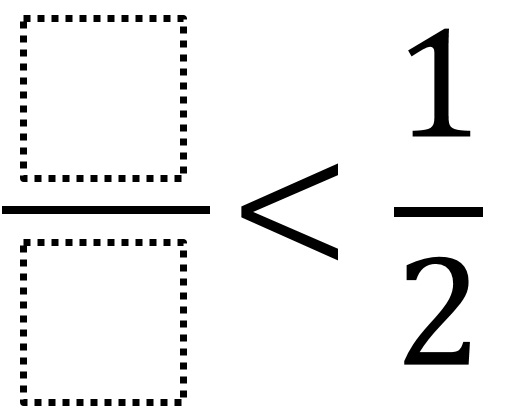Home > Grade 3 > Fractions Less Than One Half

# Fractions Less Than One Half

Directions: Using the digits 1 to 9 at most one time each, fill in the boxes to create as many fractions as possible that are less than one half.### Hint

How can you tell if a fraction is less than one half?
What patterns do you notice?
How can you be certain you have all possible solutions?

### Answer

16 fractions less than one half
1/9, 2/9, 3/9, 4/9, 1/8, 2/8, 3/8, 1/7, 2/7, 3/7, 1/6, 2/6, 1/5, 2/5, 1/4, 1/3

Source: Christine Newell

## Equivalent Fractions

Directions: Use the digits 1 to 9, at most one time each, to make three …

### 2 comments

1.I’m curious as to why you are counting equivalent fractions separately in your answer. Since 2/8 = 1/4 and 3/9 = 2/6 = 1/3, I initially found only 13 fractions.

•Just seeing this. I would count them as separate answers because although they are equivalent, they have a “different number and size of parts” which is language that comes from a 4th grade standard. A question on the equivalent fractions students find would be a great follow-up question, though!